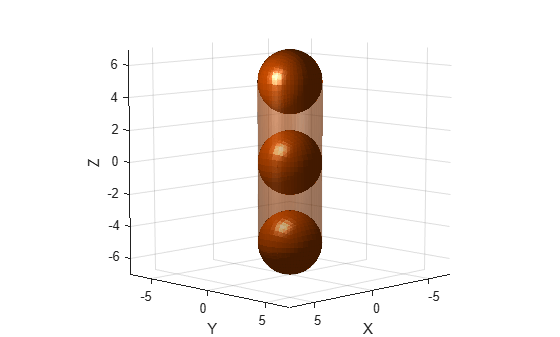# genspheres

Generate spheres along central line segment of capsule

Since R2022b

## Syntax

``spheres = genspheres(capsule,ratio)``

## Description

example

````spheres = genspheres(capsule,ratio)` generates spheres along the central line segment of the collision capsule `capsule` at the specified normalized positions `ratio` of the line segment.```

## Examples

collapse all

Create a collision capsule with a radius of `2` and length of `10`. Visualize the capsule.

```cCapsule = collisionCapsule(2,10); [~,p] = show(cCapsule);```

Generate spheres at ratios `0.0`, `0.5`, and `1.0` of the capsule length.

`spheres = genspheres(cCapsule,linspace(0,1,3));`

Display the positions of the spheres.

```for i = 1:length(spheres) disp(tform2trvec(spheres{i}.Pose)) end```
``` 0 0 -5 0 0 0 0 0 5 ```

Set the face and edge alphas of the capsule to low values. This ensures that both the spheres are visible when you add them to the figure.

```p.FaceAlpha = 0.4; p.EdgeAlpha = 0.01; hold on```

Display the generated spheres on the capsule.

`cellfun(@show,spheres);`## Input Arguments

collapse all

Collision capsule, specified as a `collisionCapsule` object.

Example: `collisionCapsule(3,5)`

Normalized positions along the central line segment of the collision capsule, specified as an N-element row vector of values in the range [0, 1]. N is the number of collision spheres to generate. Each element specifies the position of a sphere as a percentage of the central segment length.

Example: For collision capsule with a central line segment length of 4 meters, a ratio position vector `[0.25 0.5 0.75]` generates collision spheres at 1, 2, and 3 meters along the central line segment of the collision capsule.

## Output Arguments

collapse all

Collision spheres, returned as an N-element cell array of `collisionSphere` objects, where N is the number of generated collision spheres.

## Version History

Introduced in R2022b